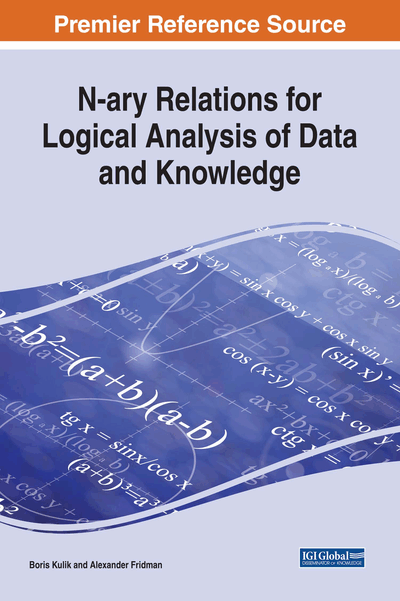# Basic Mathematical Structures

Copyright: © 2018 |Pages: 96
DOI: 10.4018/978-1-5225-2782-4.ch001
OnDemand PDF Download:
Available
\$37.50
No Current Special Offers

## Abstract

This chapter presents some conventional means of logical analysis. It is necessary to show the scope and features of our approach. In particular, we refer to algebra of sets and algebra of logic (propositional algebra), which belong to the class of Boolean algebras, as well as partially ordered sets and the theory of relations.
Chapter Preview

... If there are controversies among people, it would be sufficient just to say “Let us calculate!” in order to ... make clear who was right. – Gottfried Wilhelm Leibniz

Top

## Introduction

Here we review means of logical analysis, some of which have historically played an important role in shaping this scientific domain, while others are used in modern software systems in such areas as artificial intelligence and system analysis. These means can be divided into two categories: 1) systems based on the formal approach (i.e., TFS); 2) algebraic systems. Within TFS, in addition to classical logics (syllogistic, propositional logic, predicate logic, etc.), non-classical logics (default logic, non-monotonic logic, etc.) are actively developed. Non-classical logics are often used for modeling and analysis of defeasible reasoning (reasoning with hypotheses and abductive conclusions), but we support the opinion that it is advisable to solve this kind of computer problems algebraically, by using laws and operations of algebra of sets. Subsequent chapters are devoted to a detailed presentation of this approach to logical analysis.

Among algebraic systems, providing solutions for a wide range of logical-analysis tasks, we consider algebra of sets and algebra of logic (propositional algebra), which belong to the class of Boolean algebras, as well as partially ordered sets and theory of relations.

## Complete Chapter List

Search this Book:
Reset